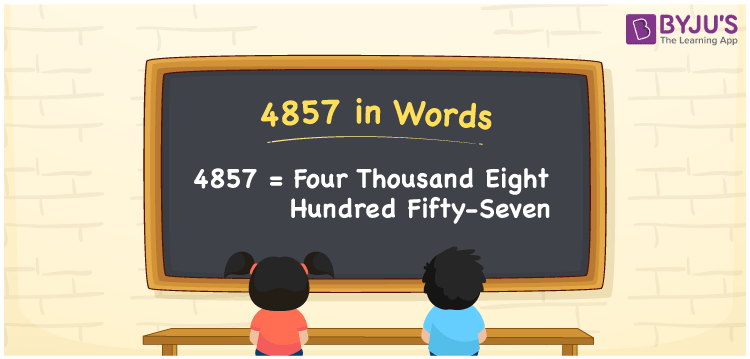# 4857 in words

4857 in words is written as Four Thousand Eight Hundred and Fifty Seven. 4857 represents the count or value. The article on Counting Numbers can give you an idea about count or counting. The number 4857 is used in expressions that relate to money, distance, length and others. Let us consider an example for 4857. ”This botanical garden has more than Four Thousand Eight Hundred and Fifty Seven types of plants.”

 4857 in words Four Thousand Eight Hundred and Fifty Seven Four Thousand Eight Hundred and Fifty Seven in Numbers 4857

## 4857 in English Words## How to Write 4857 in Words?

We can convert 4857 to words using a place value chart. The number 4857 has 4 digits, so let’s make a chart that shows the place value up to 4 digits.

 Thousands Hundreds Tens Ones 4 8 5 7

Thus, we can write the expanded form as:

4 × Thousand + 8 × Hundred + 5 × Ten + 7 × One

= 4 × 1000 + 8 × 100 + 5 × 10 + 7 × 1

= 4857

= Four Thousand Eight Hundred and Fifty Seven.

4857 is the natural number that is succeeded by 4856 and preceded by 4858.

4857 in words – Four Thousand Eight Hundred and Fifty Seven.

Is 4857 an odd number? – Yes.

Is 4857 an even number? – No.

Is 4857 a perfect square number? – No.

Is 4857 a perfect cube number? – No.

Is 4857 a prime number? – No.

Is 4857 a composite number? – Yes.

## Solved Example

1. Write the number 4857 in expanded form

Solution: 4 × 1000 + 8 × 100 + 5 × 10 + 7 × 1

We can write 4857 = 4000 + 800 + 50 + 7

= 4 × 1000 + 8 × 100 + 5 × 10 + 7 × 1

## Frequently Asked Questions on 4857 in words

Q1

### How to write 4857 in words?

4857 in words is written as Four Thousand Eight Hundred and Fifty Seven.
Q2

### Is 4857 a perfect square number?

No. 4857 is not a perfect square number.
Q3

### Is 4857 a prime number?

No. 4857 is not a prime number.